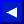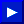The word IF is followed by an expression, which can include variables, strings, numbers, comparisons, and logical operators. The word THEN appears on the same line and is followed by either a line number or one or more BASIC statements. When the expression is false, everything after the word THEN on that line is ignored, and execution continues with the next line number in the program. A true result makes the program either branch to the line number after the word THEN or execute whatever other BASIC statements are found on that line.

EXAMPLE of IF...GOTO...Statement:
```   100 INPUT "TYPE A NUMBER"; N
110 IF N <= 0 GOTO 200
120 PRINT "SQUARE ROOT=" SQR(N)
130 GOTO 100
200 PRINT "NUMBER MUST BE >0"
210 GOTO 100
```

This program prints out the square root of any positive number. The IF statement here is used to validate the result of the INPUT. When the result of N <= 0 is true, the program skips to line 200, and when the result is false the next line to be executed is 120. Note that THEN GOTO is not needed with IF...THEN, as in line 110 where GOTO 200 actually means THEN GOTO 200.

EXAMPLE OF IF...THEN...Statement:
```   100 FOR L = 1 TO 100
110 IF RND(1) < .5 THEN X=X+1: GOTO 130
120 Y=Y+1
130 NEXT L
140 PRINT "HEADS=" X
150 PRINT "TAILS= " Y
```

The IF in line 110 tests a random number to see if it is less than .5. When the result is true, the whole series of statements following the word THEN are executed: first X is incremented by 1, then the program skips to line 130. When the result is false, the program drops to the next statement, line 120.This page has been created by Sami Rautiainen. Read the small print. Last updated November 14, 1998.